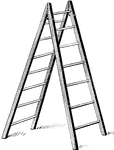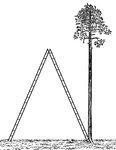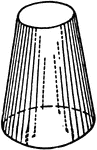### Prismatoid With 2 Circular Bases

An illustration of a prismatoid with 2 circular bases.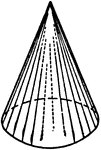### Prismatoid With Circular Base

An illustration of a prismatoid with circular base.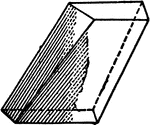An illustration of a prismatoid with faces that are quadrilaterals.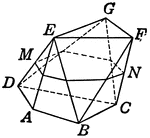### Prismatoid With Triangular Faces

An illustration of a prismatoid with triangular faces and points labeled.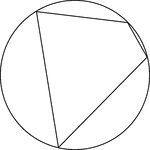Illustration of a cyclic quadrilateral, a quadrilateral inscribed in a circle. This can also be described…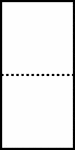### Divided Rectangle

A rectangle divided evenly by a dotted line.### Regular Heptagon/Septagon Circumscribed about a Circle

Illustration of a regular heptagon/septagon circumscribed about a circle. This can also be described…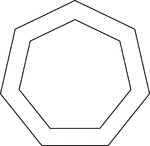### 2 Concentric Septagons/Heptagons

Illustration of 2 regular concentric heptagons/septagons.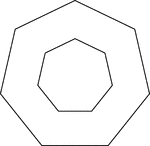### 2 Concentric Septagons/Heptagons

Illustration of 2 regular concentric heptagons/septagons.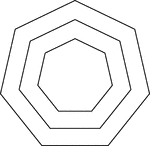### 3 Concentric Septagons/Heptagons

Illustration of 3 regular concentric heptagons/septagons that are equally spaced.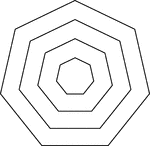### 4 Concentric Septagons/Heptagons

Illustration of 4 regular concentric heptagons/septagons that are equally spaced.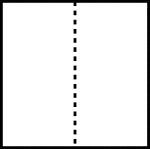### Divided Square

A square divided evenly by a dotted line.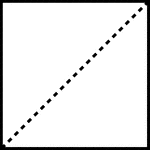### Divided Square

A square divided evenly by a dotted line, known as the diagonal.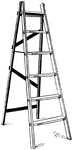Illustration of a stepladder that is opened to form an isosceles triangle with the ground.